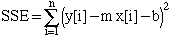Name: Murray Who is asking: Student Level: Secondary Question: If you have a set of coordinates (x,y),(x,y),...,(x[n],y[n]),find the value of m and b for which SIGMA[from 1 to m=n]AbsoluteValue(y[m]-m*x[m]-b) is at its absolute minimum. Hi Murray, The usual Linear Regression method is to minimize the sum of squares, that is to minimizeThere are theoretical and practical reasons for using the sum of squares rather than the sum of the absolute values. One of the practical reasons is that the the power of calculus can be used to find the values of m and b that minimize SSE. I don't know of an expression for the values of m and b that minimize the sum of the absolute values. Cheers, Harley Go to Math Central